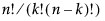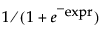Converts the temperature n to the value of explanatory variable in Arrhenius model.
ea.
`e()^a`
Returns a more accurate calculation of Exp(x)-1 when x is very small.
Fit Transform To Normal(Distribution("name"), Y(vector), <Freq(vector))
The gamma function of x, or for each row if x is a column:
Gamma(t, limit) is the same integral as Gamma(t) but with the limit of integration that is defined instead of infinity.
Returns the log gamma function for t, which is the natural log of gamma.
LnZ() is intended for internal use by derivatives. The Ln(n) function is recommended instead.
Returns the natural logarithm (base e logarithm) of n. An optional second argument lets you specify a different base. For example, Log(n,3) for the base 3 logarithm of n. The Log argument can be any numeric expression. The expression Log(e()) evaluates as 1, and Log(32,2) is 5.
Same as Log(1 + x), except that it is more accurate when x is very small.
Returns log(p/(1-p)).
This function returns the number of n things taken k at a time (“n choose k”) and is computed in the standard way using factorials, as. For example, NChooseK(5,2) evaluates as 10.
Raises a to the power of b.
The product of a multiplied by itself b times.
For Power(), the second argument (b) is optional, and the default value is 2. Power(a) returns a2.
Returns the rth root of n, where r defaults to 2 for square root.
Returns x1*x2*(x1-x2). This function supports notation for cubic mixture models.
Equivalent to Squash(-expr), or.
Returns the trigamma function evaluated at n. The trigamma function is the derivative of the digamma function.

Help created on 7/12/2018